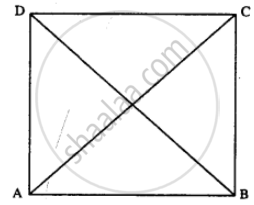# If the Diagonals of a Parallelogram Are of Equal Lengths, the Parallelogram is a Rectangle. Prove It. - Mathematics

Sum

If the diagonals of a parallelogram are of equal lengths, the parallelogram is a rectangle. Prove it.

#### SolutionGiven : //gm ABCD in which AC = BD

To Prove: ABCD is rectangle.

Proof : In ∆ABC and ∆ABD

AB = AB (Common)

AC = BD (Given)

BC = AD (opposite sides of ||gm)

∆ABC = ∆ABD (S.S.S. Rule)

∠A = ∠B

But AD // BC (opp. sides of ||gm are ||)

∠A + ∠B = 180°

∠A = ∠B = 90°

Similarly ∠D = ∠C = 90°

Hence ABCD is a rectangle.

Is there an error in this question or solution?
Chapter 17: Special Types of Quadrilaterals - Exercise 17 [Page 199]

#### APPEARS IN

Selina Concise Mathematics Class 8 ICSE
Chapter 17 Special Types of Quadrilaterals
Exercise 17 | Q 13 | Page 199
Share